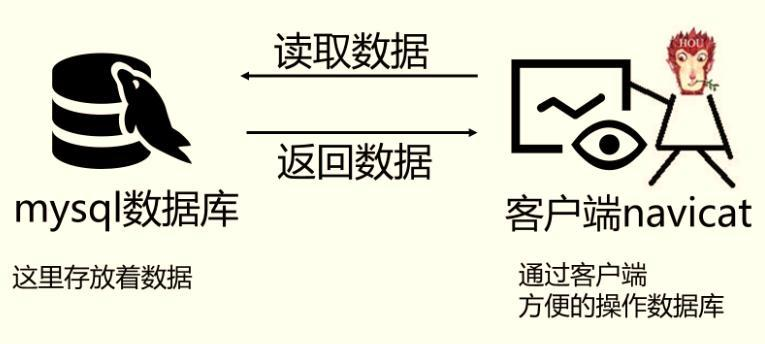ps：这些题考察SQL的编写能力，对于这类型的题目，需要你先把4张表之间的关联关系搞清楚了，最好的办法是自己在草稿纸上画出关联图，然后再编写对应的SQL语句就比较容易了。下图是我画的这4张表的关系图，可以看出它们之间是通过哪些外键关联起来的：

（如何你还不懂什么是数据库，什么是客户端navicat，可以先学习这个：

1.创建表

1）创建学生表（student）

2）创建成绩表（score）

3）创建课程表（course）

4）教师表（teacher）

2.向表中添加数据

1）向学生表里添加数据

``````insert into student(学号,姓名,出生日期,性别)
values('0001' , '猴子' , '1989-01-01' , '男');

insert into student(学号,姓名,出生日期,性别)
values('0002' , '猴子' , '1990-12-21' , '女');

insert into student(学号,姓名,出生日期,性别)
values('0003' , '马云' , '1991-12-21' , '男');

insert into student(学号,姓名,出生日期,性别)
values('0004' , '王思聪' , '1990-05-20' , '男');``````

2）成绩表（score）

``````insert into score(学号,课程号,成绩)
values('0001' , '0001' , 80);

insert into score(学号,课程号,成绩)
values('0001' , '0002' , 90);

insert into score(学号,课程号,成绩)
values('0001' , '0003' , 99);

insert into score(学号,课程号,成绩)
values('0002' , '0002' , 60);

insert into score(学号,课程号,成绩)
values('0002' , '0003' , 80);

insert into score(学号,课程号,成绩)
values('0003' , '0001' , 80);

insert into score(学号,课程号,成绩)
values('0003' , '0002' , 80);

insert into score(学号,课程号,成绩)
values('0003' , '0003' , 80);``````

3）课程表

``````insert into course(课程号,课程名称,教师号)
values('0001' , '语文' , '0002');

insert into course(课程号,课程名称,教师号)
values('0002' , '数学' , '0001');

insert into course(课程号,课程名称,教师号)
values('0003' , '英语' , '0003');``````

4）教师表里添加数据

``````-- 教师表：添加数据
insert into teacher(教师号,教师姓名)
values('0001' , '孟扎扎');

insert into teacher(教师号,教师姓名)
values('0002' , '马化腾');

-- 这里的教师姓名是空值（null）
insert into teacher(教师号,教师姓名)
values('0003' , null);

-- 这里的教师姓名是空字符串（''）
insert into teacher(教师号,教师姓名)
values('0004' , '');``````

1.简单查询

``````select count(教师号)
from teacher
where 教师姓名 like '孟%';``````

2.汇总分析

``````*

select 查询结果 [总成绩:汇总函数sum]
from 从哪张表中查找数据[成绩表score]
where 查询条件 [课程号是0002]
*/
select sum(成绩)
from score
where 课程号 = '0002';``````

``````/*

select 学号，成绩表里学号有重复值需要去掉
from 从课程表查找score;
*/
select count(distinct 学号) as 学生人数
from score;``````

``````/*

select 查询结果 [课程ID：是课程号的别名,最高分：max(成绩) ,最低分：min(成绩)]
from 从哪张表中查找数据 [成绩表score]
where 查询条件 [没有]
group by 分组 [各科成绩：也就是每门课程的成绩，需要按课程号分组];
*/
select 课程号,max(成绩) as 最高分,min(成绩) as 最低分
from score
group by 课程号;``````

``````/*

select 查询结果 [课程号，选修该课程的学生数：汇总函数count]
from 从哪张表中查找数据 [成绩表score]
where 查询条件 [没有]
group by 分组 [每门课程：按课程号分组];
*/
select 课程号, count(学号)
from score
group by 课程号;``````

``````/*

select 查询结果 [性别，对应性别的人数：汇总函数count]
from 从哪张表中查找数据 [性别在学生表中，所以查找的是学生表student]
where 查询条件 [没有]
group by 分组 [男生、女生人数：按性别分组]
having 对分组结果指定条件 [没有]
order by 对查询结果排序[没有];
*/
select 性别,count(*)
from student
group by 性别;``````

``````/*

select 查询结果 [学号，平均成绩：汇总函数avg(成绩)]
from 从哪张表中查找数据 [成绩在成绩表中，所以查找的是成绩表score]
where 查询条件 [没有]
group by 分组 [平均成绩：先按学号分组，再计算平均成绩]
having 对分组结果指定条件 [平均成绩大于60分]
*/
select 学号, avg(成绩)
from score
group by 学号
having avg(成绩)>60;``````

``````/*

select 查询结果 [学号,每个学生选修课程数目：汇总函数count]
from 从哪张表中查找数据 [课程的学生学号：课程表score]
where 查询条件 [至少选修两门课程：需要先计算出每个学生选修了多少门课，需要用分组，所以这里没有where子句]
group by 分组 [每个学生选修课程数目：按课程号分组，然后用汇总函数count计算出选修了多少门课]
having 对分组结果指定条件 [至少选修两门课程：每个学生选修课程数目>=2]
*/
select 学号, count(课程号) as 选修课程数目
from score
group by 学号
having count(课程号)>=2;``````

``````/*

1）查找出姓名相同的学生有谁，每个姓名相同学生的人数

select 查询结果 [姓名,人数：汇总函数count(*)]
from 从哪张表中查找数据 [学生表student]
where 查询条件 [没有]
group by 分组 [姓名相同：按姓名分组]
having 对分组结果指定条件 [姓名相同：count(*)>=2]
order by 对查询结果排序[没有];
*/

select 姓名,count(*) as 人数
from student
group by 姓名
having count(*)>=2;``````

``````/*

select 查询结果 [课程号]
from 从哪张表中查找数据 [成绩表score]
where 查询条件 [不及格：成绩 <60]
group by 分组 [没有]
having 对分组结果指定条件 [没有]
order by 对查询结果排序[课程号从大到小排列：降序desc];
*/
select 课程号
from score
where 成绩<60
order by 课程号 desc;``````

``````/*

select 查询结果 [课程号,平均成绩：汇总函数avg(成绩)]
from 从哪张表中查找数据 [成绩表score]
where 查询条件 [没有]
group by 分组 [每门课程：按课程号分组]
having 对分组结果指定条件 [没有]
order by 对查询结果排序[按平均成绩升序排序:asc，平均成绩相同时，按课程号降序排列:desc];
*/
select 课程号, avg(成绩) as 平均成绩
from score
group by 课程号
order by 平均成绩 asc,课程号 desc;``````

``````/*

select 查询结果 []
from 从哪张表中查找数据 [成绩表score]
where 查询条件 [课程编号为“04”且分数小于60]
group by 分组 [没有]
having 对分组结果指定条件 []
order by 对查询结果排序[查询结果按按分数降序排列];
*/
select 学号
from score
where 课程号='04' and 成绩 <60
order by 成绩 desc;``````

``````/*

select 查询结果 [要求输出课程号和选修人数]
from 从哪张表中查找数据 []
where 查询条件 []
group by 分组 [每门课程：按课程号分组]
having 对分组结果指定条件 [学生选修人数(超过2人的课程才统计)：每门课程学生人数>2]
order by 对查询结果排序[查询结果按人数降序排序，若人数相同，按课程号升序排序];
*/
select 课程号, count(学号) as '选修人数'
from score
group by 课程号
having count(学号)>2
order by count(学号) desc,课程号 asc;``````

``````/*

1）[两门以上][不及格课程]限制条件
2）[同学的学号及其平均成绩]，也就是每个学生的平均成绩，显示学号，平均成绩

1）不及格课程
2）两门以上[不及格课程]：课程数目>2

/*

select 查询结果 [学号,平均成绩：汇总函数avg(成绩)]
from 从哪张表中查找数据 [涉及到成绩：成绩表score]
where 查询条件 [没有]
group by 分组 [每个学生的平均：按学号分组]
having 对分组结果指定条件 [没有]
order by 对查询结果排序[没有];
*/
select 学号, avg(成绩) as 平均成绩
from score
group by 学号;

/*

1）不及格课程
2）两门以上[不及格课程]
select 查询结果 [学号,平均成绩：汇总函数avg(成绩)]
from 从哪张表中查找数据 [涉及到成绩：成绩表score]
where 查询条件 [限制条件：不及格课程，平均成绩<60]
group by 分组 [每个学生的平均：按学号分组]
having 对分组结果指定条件 [限制条件：课程数目>2,汇总函数count(课程号)>2]
order by 对查询结果排序[没有];
*/
select 学号, avg(成绩) as 平均成绩
from score
where 成绩 <60
group by 学号
having count(课程号)>=2;``````

3.复杂查询

``````【知识点】子查询

1.翻译成大白话
1）查询结果：学生学号，姓名
2）查询条件：所有课程成绩 < 60 的学生，需要从成绩表里查找，用到子查询

select 查询结果[学号]
from 从哪张表中查找数据[成绩表：score]
where 查询条件[成绩 < 60]
group by 分组[没有]
having 对分组结果指定条件[没有]
order by 对查询结果排序[没有]
limit 从查询结果中取出指定行[没有];

select 学号
from student
where 成绩 < 60;

select 查询结果[学号,姓名]
from 从哪张表中查找数据[学生表:student]
where 查询条件[用到运算符in]
group by 分组[没有]
having 对分组结果指定条件[没有]
order by 对查询结果排序[没有]
limit 从查询结果中取出指定行[没有];
*/
select 学号,姓名
from student
where  学号 in (
select 学号
from student
where 成绩 < 60
);``````

``````/*

【考察知识点】in，子查询
*/
select 学号,姓名
from student
where 学号 in(
select 学号
from score
group by 学号
having count(课程号) < (select count(课程号) from course)
);``````

``````select 学号,姓名
from student
where 学号 in(
select 学号
from score
group by 学号
having count(课程号)=2
);``````

1990年出生的学生名单

``````/*

*/
select 学号,姓名
from student
where year(出生日期)=1990;
``````

sql面试题：topN问题mp.weixin.qq.com4.多表查询

``````selecta.学号,a.姓名,count(b.课程号) as 选课数,sum(b.成绩) as 总成绩
from student as a left join score as b
on a.学号 = b.学号
group by a.学号;``````

``````select a.学号,a.姓名, avg(b.成绩) as 平均成绩
from student as a left join score as b
on a.学号 = b.学号
group by a.学号
having avg(b.成绩)>85;``````

``````select a.学号, a.姓名, c.课程号,c.课程名称
from student a inner join score b on a.学号=b.学号
inner join course c on b.课程号=c.课程号;``````

``````-- 考察case表达式
select 课程号,
sum(case when 成绩>=60 then 1
else 0
end) as 及格人数,
sum(case when 成绩 <  60 then 1
else 0
end) as 不及格人数
from score
group by 课程号;``````

``````-- 考察case表达式
select a.课程号,b.课程名称,
sum(case when 成绩 between 85 and 100
then 1 else 0 end) as '[100-85]',
sum(case when 成绩 >=70 and 成绩<85
then 1 else 0 end) as '[85-70]',
sum(case when 成绩>=60 and 成绩<70
then 1 else 0 end) as '[70-60]',
sum(case when 成绩<60 then 1 else 0 end) as '[<60]'
from score as a right join course as b
on a.课程号=b.课程号
group by a.课程号,b.课程名称;``````

``````select a.学号,a.姓名
from student  as a inner join score as b on a.学号=b.学号
where b.课程号='0003' and b.成绩>80;``````

【面试题类型总结】这类题目属于行列如何互换，解题思路如下：

sql面试题：行列如何互换？mp.weixin.qq.com